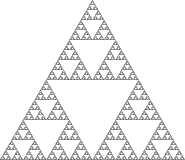# So what’s a fractal ?

posted in: Uncategorized |

So here you’ll understand what’s a fractal !A fractal is a never-ending pattern. Fractals are infinitely complex patterns that are self-similar across different scales. They are created by repeating a simple process over and over in an ongoing feedback loop. Driven by recursion, fractals are images of dynamic systems – the pictures of Chaos. Geometrically, they exist in between our familiar dimensions. Fractal patterns are extremely familiar, since nature is full of fractals. For instance: trees, rivers, coastlines, mountains, clouds, seashells, hurricanes, etc. Abstract fractals – such as the Mandelbrot Set – can be generated by a computer calculating a simple equation over and over.

# Fractal

For other uses, see Fractal (disambiguation).Mandelbrot set: Self-similarityillustrated by image enlargements. This panel, no magnification.The same fractal as above, magnified 6-fold. Same patterns reappear, making the exact scale being examined difficult to determine.The same fractal as above, magnified 100-fold.The same fractal as above, magnified 2000-fold, where the Mandelbrot set fine detail resembles the detail at low magnification.

A fractal is a natural phenomenon or a mathematical set that exhibits a repeating pattern that displays at every scale. It is also known as expanding symmetry or evolving symmetry. If the replication is exactly the same at every scale, it is called a self-similar pattern. An example of this is the Menger Sponge. Fractals can also be nearly the same at different levels. This latter pattern is illustrated in the small magnifications of the Mandelbrot set.Fractals also include the idea of a detailed pattern that repeats itself.:166; 18

Fractals are different from other geometric figures because of the way in which they scale. Doubling the edge lengths of a polygon multiplies its area by four, which is two (the ratio of the new to the old side length) raised to the power of two (the dimension of the space the polygon resides in). Likewise, if the radius of a sphere is doubled, its volume scales by eight, which is two (the ratio of the new to the old radius) to the power of three (the dimension that the sphere resides in). But if a fractal’s one-dimensional lengths are all doubled, the spatial content of the fractal scales by a power that is not necessarily an integer. This power is called the fractal dimension of the fractal, and it usually exceeds the fractal’s topological dimension.

As mathematical equations, fractals are usually nowhere differentiable. An infinite fractal curve can be conceived of as winding through space differently from an ordinary line, still being a 1-dimensional line yet having a fractal dimension indicating it also resembles a surface.:15:48Sierpinski carpet (to level 6), a two-dimensional fractal

The mathematical roots of the idea of fractals have been traced throughout the years as a formal path of published works, starting in the 17th century with notions of recursion, then moving through increasingly rigorous mathematical treatment of the concept to the study of continuous but not differentiablefunctions in the 19th century by the seminal work of Bernard Bolzano, Bernhard Riemann, and Karl Weierstrass, and on to the coining of the word fractal in the 20th century with a subsequent burgeoning of interest in fractals and computer-based modelling in the 20th century. The term “fractal” was first used by mathematician Benoît Mandelbrot in 1975. Mandelbrot based it on the Latin frāctus meaning “broken” or “fractured”, and used it to extend the concept of theoretical fractional dimensions to geometric patterns in nature.:405

There is some disagreement amongst authorities about how the concept of a fractal should be formally defined. Mandelbrot himself summarized it as “beautiful, damn hard, increasingly unuseful. That’s fractals.” The general consensus is that theoretical fractals are infinitely self-similar, iterated, and detailed mathematical constructs having fractal dimensions, of which many examples have been formulated and studied in great depth. Fractals are not limited to geometric patterns, but can also describe processes in time. Fractal patterns with various degrees of self-similarity have been rendered or studied in images, structures and sounds and found in nature, technology, art, and law. Fractals are of particular relevance in the field of chaos theory, since the graphs of most chaotic processes are fractal. Fractals are also observed from space here.http://paulbourke.net/fractals/googleearth/